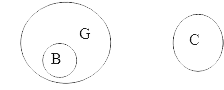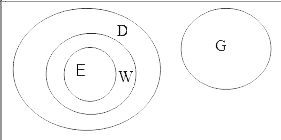# Mahindra & Mahindra Reasoning Question

NOTE: This section is not present in this company's placement process. If you still want to practise, some questions are provided below.

DIRECTIONS for the question 1 to 3: Following questions are based on five words given below:
MET ARK MOB SKI URN
(The new words formed after performing the mentioned operations may or may not necessarily be meaningful English words)
1. If the second alphabet in each of the words is changed to the previous alphabet in the English alphabetical order, how many words having no vowels will be formed?
1. None
2. One
3. Two
4. Three
5. Four
Applying the given conditions, the words will become MDT, AQK, MNB, SJI, UQN.
So, two numbers have no vowel in them.
Hence, the correct answer is option C.
2. If in each of the words, all the alphabets are arranged in English alphabetical order within the word, how many words will begin with a vowel ?
1. None
2. One
3. Two
4. Three
5. More than 3
Applying the given conditions, new words will be EMT, AKR, BMO, IKS, NRU.
Hence, the correct answer is option D
3. If the given words are arranged in the order as they would appear in a dictionary from left to right, which of the following will be second from the right ?
1. MET
2. ARK
3. MOB
4. SKI
5. URN
Applying the given conditions, the order will be ARK, MET, MOB, SKI, URN.
So, the correct answer is SKI i.e. option D.
DIRECTIONS for the question 4-5: In each question below are two/three statements followed by two conclusions numbered I and II. You have to take the two/three given statements to be true even if they seem to be at variance from commonly known facts and then decide which of the given conclusions logically follows from the given statements disregarding commonly known facts.
Give answer (1) if only conclusion I follows.
Give answer (2) if only conclusion II follows.
Give answer (3) if either conclusion I or conclusion II follows.
Give answer (4) if neither conclusion I nor conclusion II follows.
Give answer (5) if both conclusion I and conclusion II follows.
4. Statements: All bottles are glasses. No cup is a glass.
Conclusions: I. No bottle is a cup
II. Atleast some glasses are bottles
1. only conclusion I follows.
2. only conclusion II follows.
3. either conclusion I or conclusion II follows.
4. neither conclusion I nor conclusion II follows.
5. both conclusion I and conclusion II follows.clearly, both the given conclusions are true.
5. Statements: All windows are doors. All entrances are windows. No gate is a door.
Conclusions: I. Atleast some windows are gates
II. No gate is an entrance
1. only conclusion I follows.
2. only conclusion II follows.
3. either conclusion I or conclusion II follows.
4. neither conclusion I nor conclusion II follows.
5. both conclusion I and conclusion II follows.Here, "Atleast some windows are gates" is not true. And, "no gate is entrance" is true. Thus, only second conclusion is true.
DIRECTIONS for the question 6-7: Study the following information to answer the given questions:
Eight people are sitting in two parallel rows containing four people each, in such a way that there is an equal distance between adjacent persons. In row 1- A, B, C and D are seated (but not necessarily in the same order) and all of them are facing south. In row 2 - E, F, G and H are seated (but not necessarily in the same order) and all of them are facing north. Therefore, in the given seating arrangement each member seated in a row faces another member of the other row.
G sits second to right of E. The one who faces E sits to the immediate right of C. A faces the immediate neighbour of E. H sits to immediate left of the person who faces D. H does not sit at an extreme end of the line.
6. Who amongst the following faces A ?
1. E
2. F
3. G
4. H
5. Cannot be determined
We have,
G sits second to right of E i.e. _ E _ G
The one who faces E sits to the immediate right of C i.e. C _ _ _
_ E _ G A faces the immediate neighbour of E i.e. C _ A _
_ E _ G
H does not sit at an extreme end of the line i.e.
C _ A _
_ E H G
H sits to immediate left of the person who faces D i.e. C _ A D
F E H G
Pattern is 1st row C B A D.
2nd row F E H G
So, A faces H.
7. Who amongst the following faces the person who sits exactly between G and E ?
1. A
2. B
3. C
4. D
5. Cant Determine
We have,
G sits second to right of E i.e. _ E _ G
The one who faces E sits to the immediate right of C i.e. C _ _ _
_ E _ G
A faces the immediate neighbour of E i.e. C _ A _
_ E _ G
H does not sit at an extreme end of the line i.e.
C _ A _
_ E H G
H sits to immediate left of the person who faces D i.e. C _ A D
F E H G
Pattern is 1st row C B A D
2nd row F E H G
H is between G and E and H faces A.
Thus the answer will be A, the first option
DIRECTIONS for the question 8-9: Solve the following question and mark the best possible option.
Give answer (1) if only conclusion I follows.
Give answer (2) if only conclusion II follows.
Give answer (3) if either conclusion I or conclusion II follows.
Give answer (4) if neither conclusion I nor conclusion II follows.
Give answer (5) if both conclusion I and conclusion II follows.
8. Statements: G ≤ F = L ≤ J; J ≤ K = H
Conclusions: I. H = G II. G < H
1. only conclusion I follows.
2. only conclusion II follows.
3. either conclusion I or conclusion II follows.
4. neither conclusion I nor conclusion II follows.
5. both conclusion I and conclusion II follows.
From the given statements, it can be concluded that G = F = L = J = K = H.
Now it is very much clear from this that either G = H or G < H.
Thus, according to above, the answer will be option 3.
9. Statements: P < R < S < T > U
Conclusions: I. U < R II. T > P
1. only conclusion I follows.
2. only conclusion II follows.
3. either conclusion I or conclusion II follows.
4. neither conclusion I nor conclusion II follows.
5. both conclusion I and conclusion II follows.